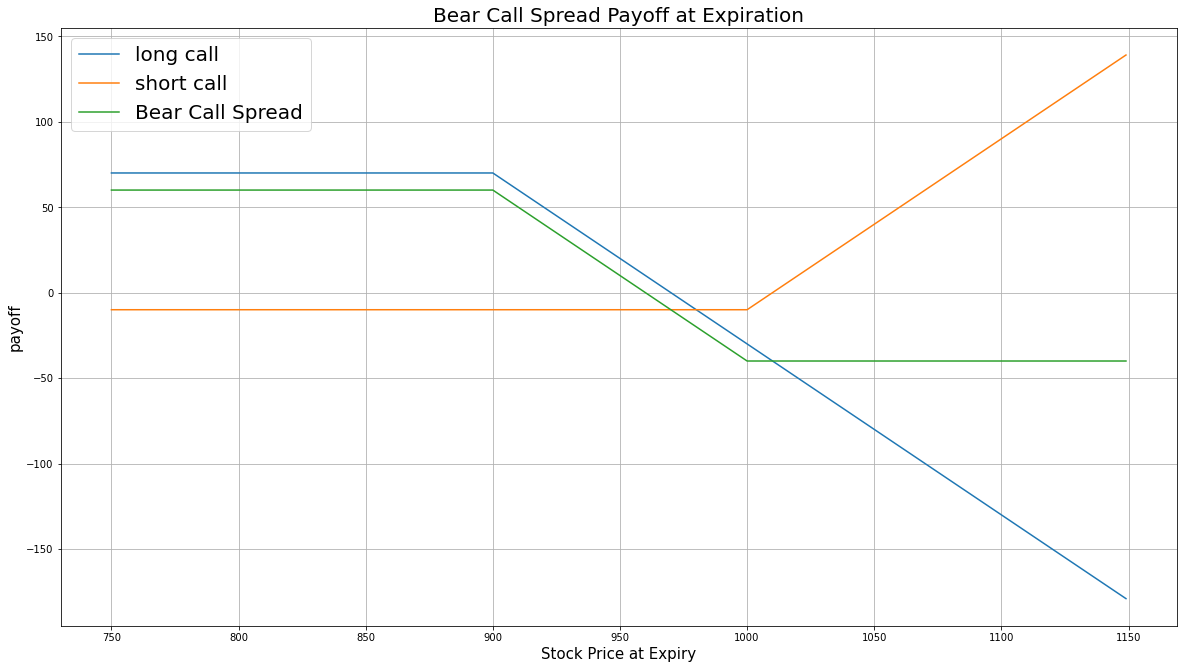# Option Strategies

### Introduction

Bear call spread, also known as short call spread, consists of selling an ITM call and buying an OTM call. Both calls have the same underlying Equity and the same expiration date. The ITM call serves as a hedge for the OTM call. The bear call spread profits from a drop in underlying asset price.

### Implementation

1. In the Initialize method, set the start date, end date, cash, and Option universe.
2. private Symbol _symbol;

public override void Initialize()
{
SetStartDate(2017, 2, 1);
SetEndDate(2017, 3, 5);
SetCash(500000);

_symbol = option.Symbol;
option.SetFilter(universe => universe.IncludeWeeklys()
.Strikes(-15, 15)
.Expiration(TimeSpan.FromDays(0), TimeSpan.FromDays(31)));
}
def Initialize(self) -> None:
self.SetStartDate(2017, 2, 1)
self.SetEndDate(2017, 3, 5)
self.SetCash(500000)

self.symbol = option.Symbol
option.SetFilter(self.UniverseFunc)

def UniverseFunc(self, universe: OptionFilterUniverse) -> OptionFilterUniverse:
return universe.IncludeWeeklys().Strikes(-15, 15).Expiration(timedelta(0), timedelta(31))
3. In the OnData method, select the expiration and strikes of the contracts in the strategy legs.
4. public override void OnData(Slice slice)
{
if (Portfolio.Invested) return;

// Get the OptionChain
var chain = slice.OptionChains.get(_symbol, null);
if (chain.Count() == 0) return;

// Select the call Option contracts with the furthest expiry
var expiry = chain.OrderByDescending(x => x.Expiry).First().Expiry;
var calls = chain.Where(x => x.Expiry == expiry && x.Right == OptionRight.Call);
if (calls.Count() == 0) return;

// Select the ITM and OTM contract strike prices from the remaining contracts
var callStrikes = calls.Select(x => x.Strike).OrderBy(x => x);
var itmStrike = callStrikes.First();
var otmStrike = callStrikes.Last();
def OnData(self, slice: Slice) -> None:
if self.Portfolio.Invested: return

# Get the OptionChain
chain = slice.OptionChains.get(self.symbol, None)
if not chain: return

# Get the furthest expiry date of the contracts
expiry = sorted(chain, key = lambda x: x.Expiry, reverse=True).Expiry

# Select the call Option contracts with the furthest expiry
calls = [i for i in chain if i.Expiry == expiry and i.Right == OptionRight.Call]
if len(calls) == 0: return

# Select the ITM and OTM contract strike prices from the remaining contracts
call_strikes = sorted([x.Strike for x in calls])
itm_strike = call_strikes
otm_strike = call_strikes[-1]
5. In the OnData method, call the OptionStrategies.BearCallSpread method and then submit the order.
6. var optionStrategy = OptionStrategies.BearCallSpread(_symbol, itmStrike, otmStrike, expiry);
Buy(optionStrategy, 1);
option_strategy = OptionStrategies.BearCallSpread(self.symbol, itm_strike, otm_strike, expiry)
self.Buy(option_strategy, 1)

Option strategies synchronously execute by default. To asynchronously execute Option strategies, set the asynchronous argument to Falsefalse. You can also provide a tag and order properties to the Buy method.

Buy(optionStrategy, quantity, asynchronous, tag, orderProperties);

self.Buy(option_strategy, quantity, asynchronous, tag, order_properties)


### Strategy Payoff

The bear call spread is a limited-reward-limited-risk strategy. The payoff is

$$\begin{array}{rcll} C^{OTM}_T & = & (S_T - K^{OTM})^{+}\\ C^{ITM}_T & = & (S_T - K^{ITM})^{+}\\ P_T & = & (C^{OTM}_T - C^{ITM}_T + C^{ITM}_0 - C^{OTM}_0)\times m - fee\\ \end{array}$$ $$\begin{array}{rcll} \textrm{where} & C^{OTM}_T & = & \textrm{OTM call value at time T}\\ & C^{ITM}_T & = & \textrm{ITM call value at time T}\\ & S_T & = & \textrm{Underlying asset price at time T}\\ & K^{OTM} & = & \textrm{OTM call strike price}\\ & K^{ITM} & = & \textrm{ITM call strike price}\\ & P_T & = & \textrm{Payout total at time T}\\ & C^{ITM}_0 & = & \textrm{ITM call value at position opening (debit paid)}\\ & C^{OTM}_0 & = & \textrm{OTM call value at position opening (credit received)}\\ & m & = & \textrm{Contract multiplier}\\ & T & = & \textrm{Time of expiration} \end{array}$$

The following chart shows the payoff at expiration:The maximum profit is the net credit you receive from opening the trade, $C^{ITM}_0 - C^{OTM}_0$. If the price declines, both calls expire worthless.

The maximum loss is $K^{OTM} - K^{ITM} + C^{ITM}_0 - C^{OTM}_0$, which occurs when the underlying price is above the strike prices of both call Option contracts.

If the Option is American Option, there is a risk of early assignment on the sold contract.

### Example

The following table shows the price details of the assets in the algorithm:

AssetPrice ($)Strike ($)
OTM call4.40835.00
ITM call36.80767.50
Underlying Equity at expiration829.08-

Therefore, the payoff is

$$\begin{array}{rcll} C^{OTM}_T & = & (S_T - K^{OTM})^{+}\\ & = & (829.08-835.00)^{+}\\ & = & 0\\ C^{ITM}_T & = & (S_T - K^{ITM})^{+}\\ & = & (829.08-767.50)^{+}\\ & = & 61.58\\ P_T & = & (C^{OTM}_T - C^{ITM}_T + C^{ITM}_0 - C^{OTM}_0)\times m - fee\\ & = & (0-61.58+36.80-4.40)\times100-1.00\times2\\ & = & -2920\\ \end{array}$$

So, the strategy losses \$2,920.

The following algorithm implements a bear call spread Option strategy:

You can also see our Videos. You can also get in touch with us via Discord.